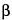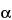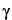Monensin Sodium
(moe nen' sin soe' dee um).
C36H61NaO11 (monensin A sodium)692.88
C35H59NaO11 (monensin B sodium)678.85
C37H63NaO11 (monensin C sodium)706.91

Monensin, sodium salt.
Stereoisomer of 2-[2-ethyloctahydro-3¢-methyl-5¢-[tetrahydro-6-hydroxy-6-(hydroxymethyl)-3,5-dimethyl-2H-pyran-2-yl][2,2¢-bifuran-5-yl]]-9-hydroxy--methoxy-,,2,8-tetramethyl-1,6-dioxaspiro[4.5]decan-7–butanoic acid sodium salt[22373-78-0].
» Monensin Sodium has a potency of not less than 800 µg per mg.
Packaging and storage— Preserve in well-closed containers. Avoid moisture and excessive heat.
Labeling— Label it to indicate that it is for veterinary use only. Label it also to state that it is for manufacturing, processing, or repackaging.
Identification— The chromatogram of the Assay preparation obtained as directed in the Assay exhibits a major peak for monensin A and a minor peak for monensin B, the retention times of which correspond to those exhibited in the chromatogram of the Standard preparation, as obtained in the Assay.
Dry it in vacuum at 60for 3 hours: it loses not more than 4% of its weight.
Content of monensin A and B activity— Using the results of the calculations in the Assay, calculate the percentage of monensin A activity in the Monensin Sodium under test by the formula:
100A / P
in which A is the potency, in mg per g, of monensin A in the Monensin Sodium under test, as determined in the Assay, and P is the potency, in mg of monensin, in each g of the Monensin Sodium under test, as determined in the Assay: not less than 90% is found. Calculate the percentage of monensin A activity plus monensin B activity in the Monensin Sodium under test by the formula:
100(A + B) / P
in which B is the potency, in mg per g, of monensin B in the Monensin Sodium under test, as determined in the Assay, and the other terms are as defined above: not less than 95% is found.
Assay—
Mobile phase , Neutralized methanol, Diluent, Derivatizing reagent, Standard preparation, Resolution solution, and Chromatographic system—Proceed as directed in the Assay under Monensin.
Assay preparation— Transfer about 100 mg of Monensin Sodium, accurately weighed, to a 100-mL volumetric flask, dissolve in and dilute with methanol to volume. If necessary, to achieve complete dissolution, sonicate for about 1 minute, and mix. Dilute an accurately measured volume of this solution quantitatively with Diluent to obtain a solution containing about 20 µg of monensin per mL.
Procedure— Proceed as directed for Procedure in the Assay under Monensin. Calculate the quantity, in mg, of monensin A in each g of the Monensin Sodium taken by the formula:
(CFD / 100,000W)(rU / rS)
in which C is the concentration, in µg per mL, of monensin activity in the Standard preparation, based on the quantity of USP Monensin Sodium RS taken, its designated potency, in µg per mg, and the extent of dilution; F is the designated percentage of monensin A in USP Monensin Sodium RS; D is the dilution factor used in preparing the Assay preparation; W is the quantity, in g, of Monensin Sodium taken to prepare the Assay preparation; and rU and rS are the monensin A peak responses obtained from the Assay preparation and the Standard preparation, respectively. Calculate the quantity, in mg, of monensin B in each g of the Monensin Sodium taken by the same formula, except that rU is the monensin B peak response obtained from the Assay preparation, and rS is the monensin A peak response obtained from the Standard preparation. Calculate the quantity, in mg, of monensin C/D in each g of the Monensin Sodium taken by the same formula, except that rU is the monensin C/D peak response obtained from the Assay preparation. Calculate the potency, in mg of monensin, in each g of the Monensin Sodium taken by the formula:
A + 0.28B + 1.5C / D
in which A is the quantity, in mg, of monensin A in each g of the Monensin Sodium taken, as calculated above; B is the quantity, in mg, of monensin B in each g of the Monensin Sodium taken; and C/D is the quantity, in mg, of monensin C/D in each g of Monensin Sodium taken, as calculated above.
Auxiliary Information— Please check for your question in the FAQs before contacting USP.
Topic/Question Contact Expert Committee
Monograph Morgan Puderbaugh, B.S.
Associate Scientific Liaison
1-301-998-6833
(SM32010) Monographs - Small Molecules 3
Reference Standards RS Technical Services
1-301-816-8129
rstech@usp.org
USP35–NF30 Page 3950